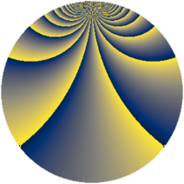# Properties

 Label 2300.2.uLevel $2300$ Weight $2$ Character orbit 2300.u Rep. character $\chi_{2300}(101,\cdot)$ Character field $\Q(\zeta_{11})$ Dimension $380$ Sturm bound $720$

# Related objects

## Defining parameters

 Level: $$N$$ $$=$$ $$2300 = 2^{2} \cdot 5^{2} \cdot 23$$ Weight: $$k$$ $$=$$ $$2$$ Character orbit: $$[\chi]$$ $$=$$ 2300.u (of order $$11$$ and degree $$10$$) Character conductor: $$\operatorname{cond}(\chi)$$ $$=$$ $$23$$ Character field: $$\Q(\zeta_{11})$$ Sturm bound: $$720$$

## Dimensions

The following table gives the dimensions of various subspaces of $$M_{2}(2300, [\chi])$$.

Total New Old
Modular forms 3780 380 3400
Cusp forms 3420 380 3040
Eisenstein series 360 0 360

## Trace form

 $$380q - 2q^{3} - 2q^{7} - 36q^{9} + O(q^{10})$$ $$380q - 2q^{3} - 2q^{7} - 36q^{9} + 6q^{11} - 2q^{13} - 13q^{17} - 7q^{19} - 23q^{21} + 28q^{23} - 23q^{27} - 5q^{29} - 13q^{31} + 5q^{33} - 50q^{37} - 42q^{39} + 24q^{41} + 24q^{43} - 50q^{47} - 116q^{49} + 38q^{51} + 14q^{53} - 53q^{57} + 26q^{59} + 40q^{61} - 59q^{63} - 8q^{67} - 157q^{69} - 131q^{71} - 32q^{73} - 35q^{77} + 50q^{79} + 10q^{81} + 11q^{83} + 64q^{87} + 46q^{89} - 30q^{91} + 10q^{93} + 41q^{97} + 201q^{99} + O(q^{100})$$

## Decomposition of $$S_{2}^{\mathrm{new}}(2300, [\chi])$$ into newform subspaces

The newforms in this space have not yet been added to the LMFDB.

## Decomposition of $$S_{2}^{\mathrm{old}}(2300, [\chi])$$ into lower level spaces

$$S_{2}^{\mathrm{old}}(2300, [\chi]) \cong$$ $$S_{2}^{\mathrm{new}}(23, [\chi])$$$$^{\oplus 9}$$$$\oplus$$$$S_{2}^{\mathrm{new}}(46, [\chi])$$$$^{\oplus 6}$$$$\oplus$$$$S_{2}^{\mathrm{new}}(92, [\chi])$$$$^{\oplus 3}$$$$\oplus$$$$S_{2}^{\mathrm{new}}(115, [\chi])$$$$^{\oplus 6}$$$$\oplus$$$$S_{2}^{\mathrm{new}}(230, [\chi])$$$$^{\oplus 4}$$$$\oplus$$$$S_{2}^{\mathrm{new}}(460, [\chi])$$$$^{\oplus 2}$$$$\oplus$$$$S_{2}^{\mathrm{new}}(575, [\chi])$$$$^{\oplus 3}$$$$\oplus$$$$S_{2}^{\mathrm{new}}(1150, [\chi])$$$$^{\oplus 2}$$